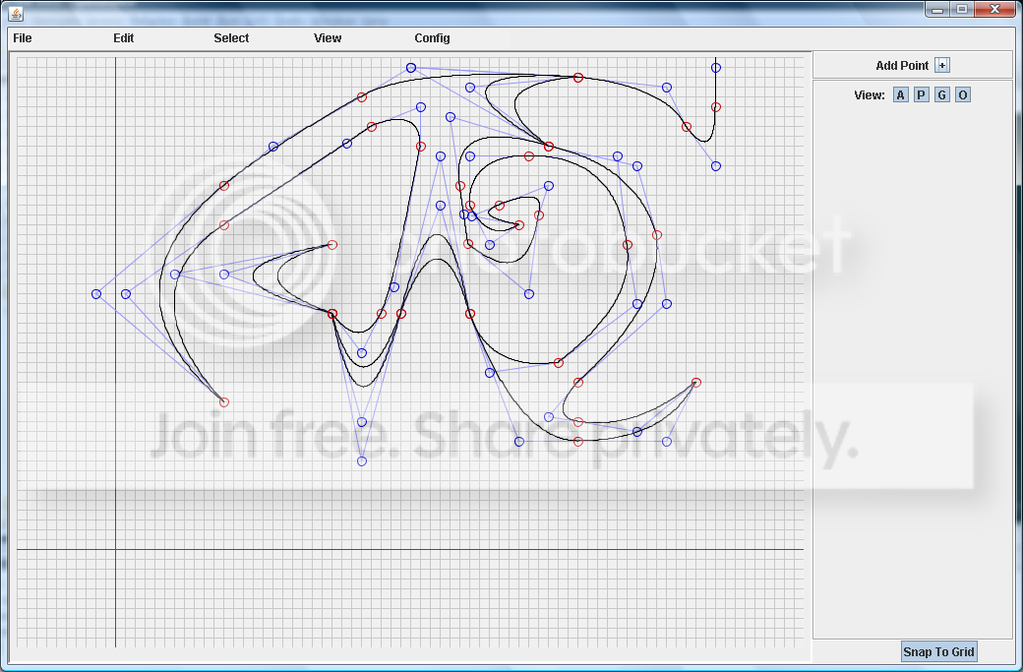-=+=- -=+=- -=+=- -=+=- -=+=- -=+=- -=+=- -=+=- -=+=- -=+=- -=+=- -=+=- -=+=- -=+=- -=+=- -=+=- -=+=- -=+=- -=+=- -=+=- -=+=- -=+=- -=+=- -=+=- -=+=- -=+=- -=+=- -=+=- -=+=- -=+=- (c) WidthPadding Industries 1987 0|448|0 -=+=- -=+=- -=+=- -=+=- -=+=- -=+=- -=+=- -=+=- -=+=- -=+=- -=+=- -=+=- -=+=- -=+=- -=+=- -=+=- -=+=- -=+=- -=+=- -=+=- -=+=- -=+=- -=+=- -=+=- -=+=- -=+=- -=+=- -=+=- -=+=- -=+=-
SoCoder -> Snippet Home -> Editors

mike_gCreated : 07 July 2008
Edited : 07 July 2008
Language : Blitz

### Spline Editor

Edit Paths Made Of Bezier Curves

I had a play around with Jay's bezier curve code and came up with the start of a simple spline editor. At the moment it only works with quadratic bezier curves, but if I find the time i plan to add cubic curves, save/load co-ords to/from files and allow users to add points. It might be useful then.

Anyway heres the code:

Click on the points to drag them around

Monday, 07 July 2008, 14:51
JayenkaiLooks good enough to me!Wednesday, 09 July 2008, 16:26
mike_gCheers Jay. I decided to convert it to Java for the editor. However I plan to add code to load the files produced in Blitz and C. Heres a screenie:Wednesday, 09 July 2008, 16:27
JayenkaiNice!!Wednesday, 09 July 2008, 23:58
shroom_monkWow! That looks pretty awesome!
Thursday, 10 July 2008, 12:21
mike_gHey, I'm glad you like it shroomHeres an effect I knocked up using a spline made in my editor:

I might have a go at coding a blitz library with different effects that work on splines, but first theres still a lot of work to do on the editor.
Tuesday, 06 April 2010, 09:51
dnaVery Clever.

Well Done